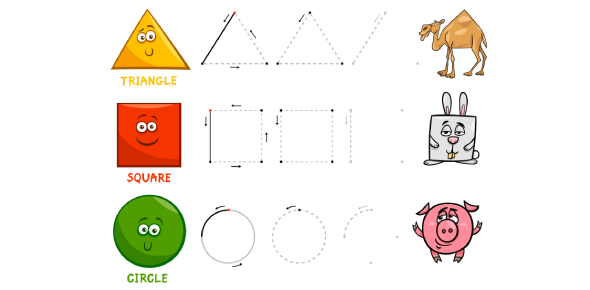# Primary 5-6 Math Quiz

5 Questions | Total Attempts: 4582SettingsThis is a quiz for primary 5 or 6 students. I hope that you'll enjoy the quiz and improve your math skills.

• 1.
189.35 + 247.68 = ?
• A.

437.03

• B.

580.33

• C.

440.23

• 2.
4x + 3 = 15 x = 18
• A.

True

• B.

False

• 3.
87.4 x 4.78 = ?
• 4.
8x = 2z 24x = 3y x + y + z = ?
• A.

44x

• B.

26x

• C.

13x

• 5.
50000mL = 50L
• A.

True

• B.

False

Related TopicsBack to top